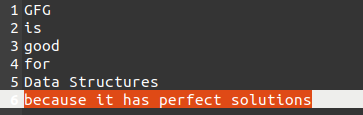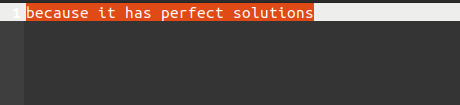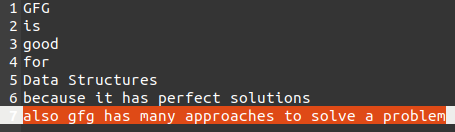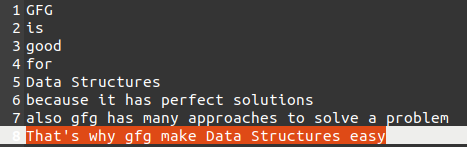Related Articles

# Add New Line to Text File in R

• Last Updated : 21 Apr, 2021

In this article, we are going to add a new line to the Text file using the R Programming language.

It can be done in different ways:

• Using write() function.
• Using lapply() function.
• Using cat() function.

Method 1: Adding text using write() function.

Now we are going to select the line which is going to be added to the text file.

`line <- "because it has perfect solutions"`

At last, we use the write() function to append the above line in the text file.

There are 3 arguments in the write() function first is the line which we want to add in the text file and that line is stored in a variable named line 2nd argument is the file name itself through this argument a connection is set between our code and the text file and the last one is the append command which is used to append the text in the text file. All these things would be seen as:

`write(line,file="Text.txt",append=TRUE)`

Below is the implementation:

## R

 `# line which we need to be add``line = ``"because it has perfect solutions"`` ` `# write function to add the file``write``(line, file = ``"Text.txt"``,``      ``append = ``TRUE``, sep = ``"\n"``)`

Output:The Red highlighted line is the line that is appended.

Note: If you put append = FALSE then the whole text file gets over-written.

## R

 `# line which we need to add on text file``line = ``"because it has perfect solutions"`` ` `# write function helps us to add text ``write``(line, file = ``"Text.txt"``, append = ``FALSE``)`

Output:Method 2: Adding text using lapply() function.

The lapply() function uses the vector to add the text to the text file. So, we create a vector of the text we want to add to the text file

`a=c("also gfg has many approaches to solve a problem")`

A vector name “a” is created who has the line we want to add to our text file.

Now we use the lapply function with the write() and anonymous function. The anonymous function is used to take each character of the vector as an argument also the append is used to append the text to the text file.

lapply(a, function(anyNameofVect){ write(anyNameofVect, file=”Text.txt”, sep=”\n”, append=TRUE, ncolumns=100000) })

Below is the implementation:

## R

 `# vector of text``a = ``c``(``"also gfg has many approaches to solve a problem"``)`` ` `# lapply function with anonymous function ``lapply``(a, ``function``(c){ ``write``(c, file = ``"Text.txt"``, ``                             ``sep = ``"\n"``, append = ``TRUE``,``                             ``ncolumns = 100000) })`

Output:Method 3: Adding text using cat() function:

The cat function simply did the same thing as the lapply. It takes the text and establish a connection with the text file using the file name and use append command to append the text to the text file

## R

 `# cat function to append the text to the text file``cat``(``"That's why gfg make Data Structures easy"``,``    ``sep = ``"\n"``, file = ``"Text.txt"``, append = ``TRUE``)`

Output:My Personal Notes arrow_drop_up